# How to use Excel text functionsMicrosoft Excel now has 14 new functions that make it easier to manipulate text within spreadsheets. Text functions can be used to display text and numbers in a more readable format – especially valuable when you’re presenting a spreadsheet to a client.

In this article, you’ll learn how to use each of Excel’s newest text functions and be provided with easy-to-follow examples. The workbook NewTextFunctions.xlsx contains our work.

Keep in mind that some functions are released to Office 365 users at different times, so you may not have them yet. To test whether you have the functions, you can try typing one (say, ‘=TEXTBEFORE) into a blank workbook. If you have the functions, follow along with the worksheet ‘OFFICE 365.’ Otherwise, refer to the worksheet ‘Not yet,’ which shows how the functions work.

## How to manipulate text with functions in Excel

Below, we’ll demonstrate how to use each of the newest text functions in Excel. Figure 1 illustrates the use of the TEXTAFTER, TEXTBEFORE, TEXTSPLIT, VSTACK, and HSTACK functions.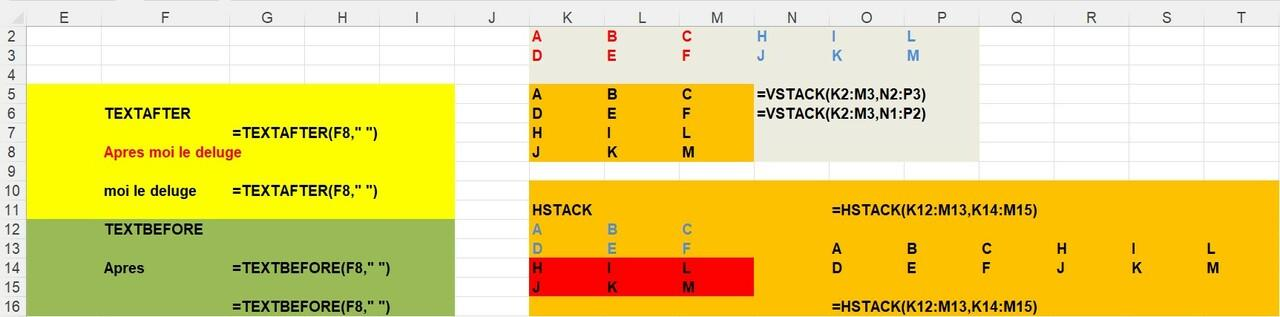Figure 1 Examples of TEXTBFORE, TEXTAFTER, TEXTSPLIT, VSTACK and HSTACK functions

1. TEXTAFTER function

The TEXTAFTER function extracts the text string from a cell that occurs after the first occurrence of a delimiter. For example, the formula =TEXTAFTER(F8," ") returns everything occurring after the first space in “Apres moi le deluge” (Louis XIV’s famous quote in which he declared, “after me, the deluge.”) The result in cell F10 is “moi le deluge.”

1. TEXTBEFORE function

The TEXTBEFORE function extracts the text string from a cell that occurs before the first occurrence of a delimiter. For example, entering the formula =TEXTBEFORE(F8," ") in cell F14 returns “Apres” in cell F14.

1. TEXTSPLIT function

The TEXTSPLIT function “splits” the contents of a cell (across multiple cells) at each occurrence of a delimiter. For example, entering the formula =TEXTSPLIT(F8," ") in cell F22 enters “Apre,” “moi,” “le” and “deluge” in separate cells. This is much better than TEXT to COLUMNS, because TEXTSPLIT will update if the original cell changes, while TEXT to COLUMNS will not.

1. VSTACK function

The VSTACK function stacks two (or more) arrays on top of each other. For example, entering the function = VSTACK(K2:M3,N2:P3) in cell K5, stacks the array

ABC
DEF

On top of the array

HIL

JKM.

1. HSTACK function

The HSTACK function horizontally stacks two (or more) arrays into one array. For example, entering the formula = HSTACK(K12:M13,K14:M15) in cell O13 yields

ABCHIL

DEFJKM.

Figure 2 illustrates the use of the TOROW and TOCOL functions.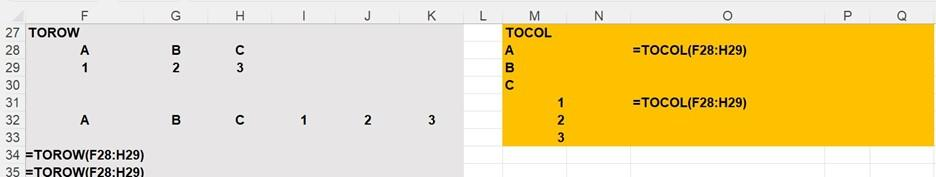Figure 2 Examples of TOROW and TOCOL functions

1. TOROW function

The TOROW function transforms an array into a single row. For example, the formula =TOROW(F28:H29) in cell F32 transforms

ABC

123

into ABC123.

1. TOCOL function

The TOCOL function transforms an array into a single column. For example, the formula = TOCOL(F28:H29) in cell M28 transforms

ABC

123

into

A

B

C

1

2

3.

Figure 3 illustrates the use of WRAPROWS and WRAPCOLS functions.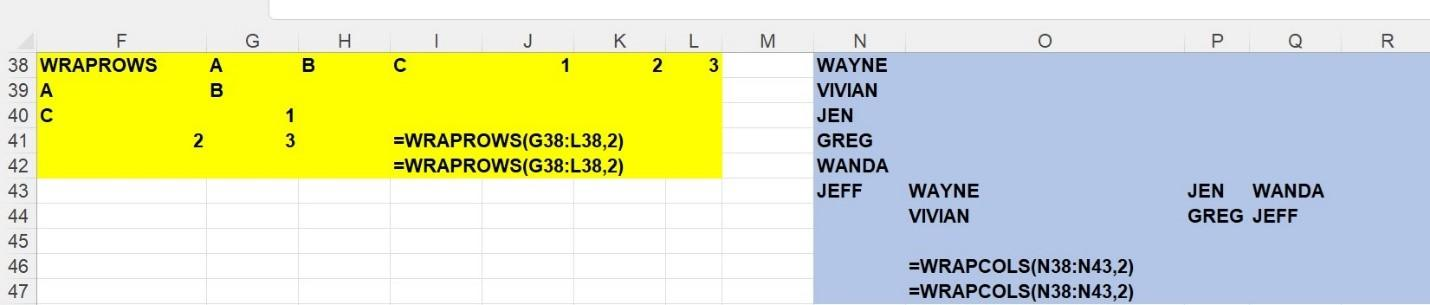Figure 3 Examples of WRAPROWS and WRAPCOLS functions

1. WRAPROWS function

The WRAPROWS function transforms a row into a designated number of rows. For example, the formula =WRAPROWS(G38:L38,2) in cell F39 transforms

 A B C 1 2 3

into rows each containing two columns. The result is

 A B C 1 2 3
1. WRAPCOLS function

The WRAPCOLS function transforms a column into a designated number of rows. The formula =WRAPCOLS(N38:N43,2) in cell O43 transforms

 WAYNE VIVIAN JEN GREG WANDA JEFF

into columns each containing two rows. The result is

 WAYNE JEN WANDA VIVIAN GREG JEFF

Figure 4 illustrates the use of the TAKE, DROP, CHOOSEROWS and CHOOSECOLS functions.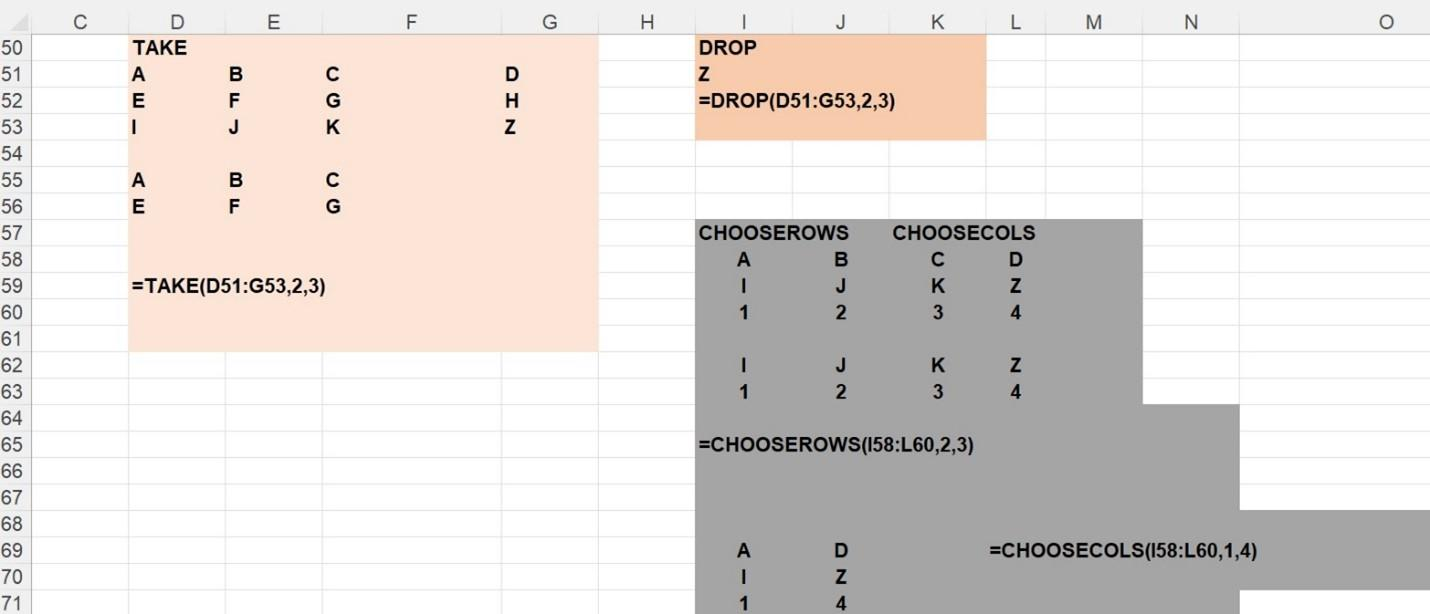Figure 4 Examples of TAKE, DROP, CHOOSEROWS, and CHOOSECOLS functions

1. TAKE function

The formula =TAKE(D51:G53,2,3) in cell D55 “takes” the first two rows and first three columns of the array

 A B C D E F G H I J K Z

yielding

 A B C E F G
1. DROP function

The formula =DROP(D51:G53,2,3) in cell I51 drops the first 2 rows and first 3 columns from the array.

 A B C D E F G H I J K Z

The result is

Z.

1. CHOOSEROWS function

The formula = CHOOSEROWS(D51:G53,1,3) in cell I58 chooses rows 1 and 3 from the array

 A B C D E F G H I J K Z

The result is the first and third rows of the initial array.

 A B C D I J K Z
1. CHOOSECOLS function

The formula = CHOOSECOLS(I58:L60,1,4) in cell I69 returns the first and fourth columns of the following.

 A B C D I J K Z 1 2 3 4

The result is the first and fourth columns of the initial array.

 A D I Z 1 4

And finally, Figure 5 illustrates the use of the EXPAND function.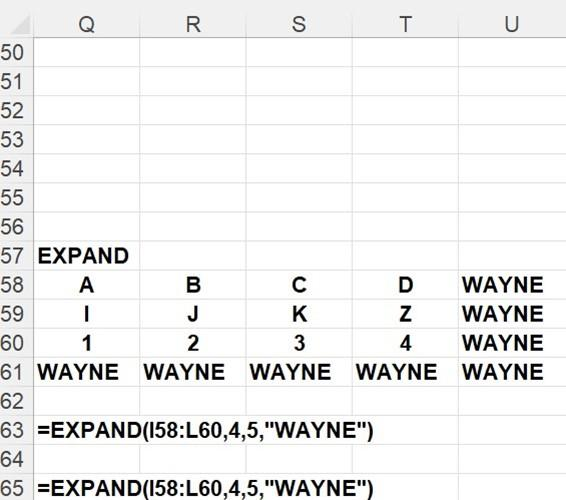Figure 5 Example of the EXPAND Function

1. EXPAND function

The formula = EXPAND(I58:L60,4,5,"WAYNE") in cell Q58 expands the array

 A B C D I J K Z 1 2 3 4

to 4 rows and 5 columns by padding the array with cells containing “WAYNE”. The result is the following.

 A B C D WAYNE I J K Z WAYNE 1 2 3 4 WAYNE WAYNE WAYNE WAYNE WAYNE WAYNE

Accountants spend countless hours using Microsoft Excel, so it’s always useful to learn new tips and tricks. For more Excel tutorials, take one of my Becker CPE courses.

More like this### Now Leaving Becker.com

You are leaving the Becker.com website. Once you click “continue,” you will be brought to a third-party website. Please be aware, the privacy policy may differ on the third-party website. Adtalem Global Education is not responsible for the security, contents and accuracy of any information provided on the third-party website. Note that the website may still be a third-party website even the format is similar to the Becker.com website.